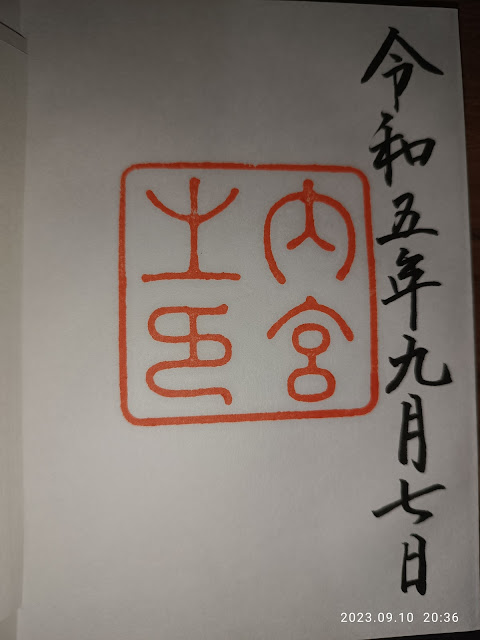### JAVA--以例子來看&、&&與 |、||的差異

public class test023  {
public static void main(String[] args) {

int a = 1, b = 2;

if (a > 1 & b++ >2){ System.out.println("Hello"); }
System.out.println("a = " + a + ", b = " + b);

a = 1 ;
b = 2 ;

if (a > 1 && b++ > 2){ System.out.println("Hello"); }
System.out.println("a = " + a + ", b = " + b);

}

a=1,b=3
a=1,b=2

「|」與「||」也是一樣的道理，測試如下：
public class test024  {
public static void main(String[] args) {

int a = 2, b = 2;

if (a > 1 | b++ >2){ System.out.println("Hello"); }
System.out.println("a = " + a + ", b = " + b);

a = 2 ;
b = 2 ;

if (a > 1 || b++ > 2){ System.out.println("Hello"); }
System.out.println("a = " + a + ", b = " + b);

}
}

### 犬山城御城印、名古城御朱印、伊勢神宮御朱印、郵便局風景印、星巴克隨行卡